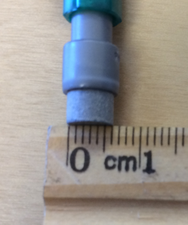# Unit Conversions

## Objective

Express decimal measurements in terms of a smaller unit.

## Common Core Standards

### Core Standards

?

• 4.MD.A.1 — Know relative sizes of measurement units within one system of units including km, m, cm; kg, g; lb, oz.; l, ml; hr, min, sec. Within a single system of measurement, express measurements in a larger unit in terms of a smaller unit. Record measurement equivalents in a two column table. For example, know that 1 ft is 12 times as long as 1 in. Express the length of a 4 ft snake as 48 in. Generate a conversion table for feet and inches listing the number pairs (1, 12), (2, 24), (3, 36), …

• 4.MD.A.2 — Use the four operations to solve word problems involving distances, intervals of time, liquid volumes, masses of objects, and money, including problems involving simple fractions or decimals, and problems that require expressing measurements given in a larger unit in terms of a smaller unit. Represent measurement quantities using diagrams such as number line diagrams that feature a measurement scale.

?

• 3.MD.A.1

• 3.MD.A.2

• 4.NF.C.5

• 4.NF.C.6

## Criteria for Success

?

1. Understand that 0.1 centimeter is equivalent to a millimeter.
2. Understand that 0.01 meter is equivalent to a centimeter.
3. Convert metric measurements from a larger unit written as a decimal to a smaller unit.

## Tips for Teachers

?

#### Remote Learning Guidance

If you need to adapt or shorten this lesson for remote learning, we suggest prioritizing Anchor Task 2 (benefits from worked example). Find more guidance on adapting our math curriculum for remote learning here.

#### Fishtank Plus

• Problem Set
• Student Handout Editor
• Vocabulary Package

?

### Problem 1

Ms. Cole measures the width of her pencil eraser. She says it is 0.6 cm wide. Mr. Duffy says it is 6 mm wide. Who is correct? Explain your answer.### Problem 2

Solve. Show or explain your work.

a. 0.01 m = ________ cm

b. 0.06 m = ________ mm

c. 0.3 L = ________ mL

d. 0.83 kg = ________ g

e. 10.42 km = ________ m

## Problem Set & Homework

#### Discussion of Problem Set

• How can knowing that 10 cm is equal to 100 mm help you check to make sure your answer to #4(c) is reasonable?
• Which cereal should Mr. Silver buy in #5? Why?
• How can knowing that 1 m is equal to 1,000 mm help you check to make sure your answer to #6(a) is reasonable?
• What is the combined length of Ms. Ruggiero’s ticker tape? Is it enough to use for the name tags? Why or why not?
• When have you heard someone talk about a decimal unit before?

?

### Problem 1

Show or explain how you know that 3.5 centimeters = 35 millimeters.

### Problem 2

Solve.

 a. 4.8 meters = __________ millimeters b.  5.04 L = __________ mL c.  0.4 kg = __________ g d.  10.67 km = __________ m

### Mastery Response

?